# An Overview on Optimization Algorithms in Deep Learning 1

Published:

Recently, I have been learning about optimization algorithms in deep learning. And it is necessary, I think, to sum them up, so I plan to write a series of articles about different kinds of these algorithms. This article will mainly talk about the basic optimization algorithms used in machine learning and deep learning.

Gradient descent is the most basic gradient-based algorithm to train a deep model. Once we get the function, let’s say $L$, that needs to optimize, especially when the function is convex, we can easily apply this method to approach the minimum of a function. This method involves updating the model parameters $\theta$ with a small step in the direction of the gradient of the objective function. For the case of supervised learning with data pairs $(x^{(i)}, y^{(i)})$, we have

$\theta\leftarrow \theta + \epsilon\nabla_\theta \sum_t L(f(x^{(i)};\theta), y^{(i)};\theta).$

where $\epsilon$ is the learning rate or the step size, that controls the size of the step the parameter takes in each iteration.

In spite of its impressive convergence property, batch gradient descent is rarely used in machine learning, because the cost of calculating the sum over gradient of each sample would be enormous when the number of training samples becomes large. A computationally efficient way is stochastic gradient descent in which we use the stochastic estimator of the gradient to perform its update. Based on the assumption that all samples are i.i.d, we sample one or a small suubset of $m$ training samples and compute their gradient. Then we use the gradient to update the parameter $\theta$.

When $m=1$, this algorithm is sometimes called online gradient descent. When $m>1$, the algorithm is sometimes called minibatch SGD. The algorithm is showed below.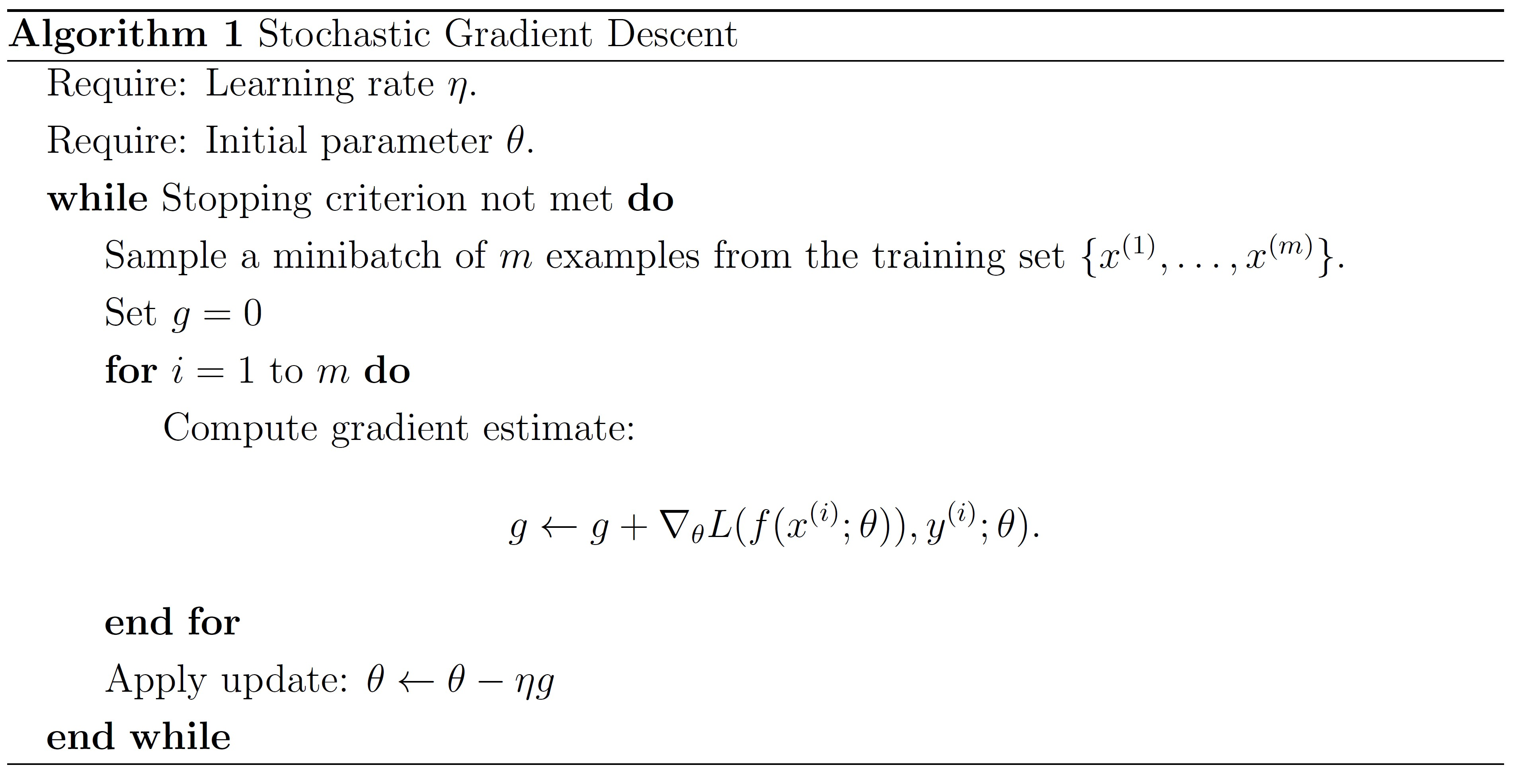GD or SGD, cannot escape from the occasion: if the learning rate is too big, the weights may travel to and fro across the ravine and not converge to the minimum; if the learning rate is too small, it will take a long time to converge or coonverge to local minima. Thus, we need adjust the learning rate accordingly: if the error is falliing fairly consistently but slowly, increase the learning rate; if the error keeps getting worse or oscillates wildly, then we should reduce the learning rate. Is there any algorithms that adapt the learning rate automatically?

## Momentum Method

One method of speeding up training is the momentum method. This is perhaps the simplest extension to SGD that has been sucessfully used for decades. The intuition behind momentum, as the name suggests, is derived from a physical interpretation of the optimization process. Imaging a ball is rolling on a slope, the track of the ball is a combination of velocity and the instantaneous force pulling the ball downhill. And the momentum plays a role in accumulating gradient contribution.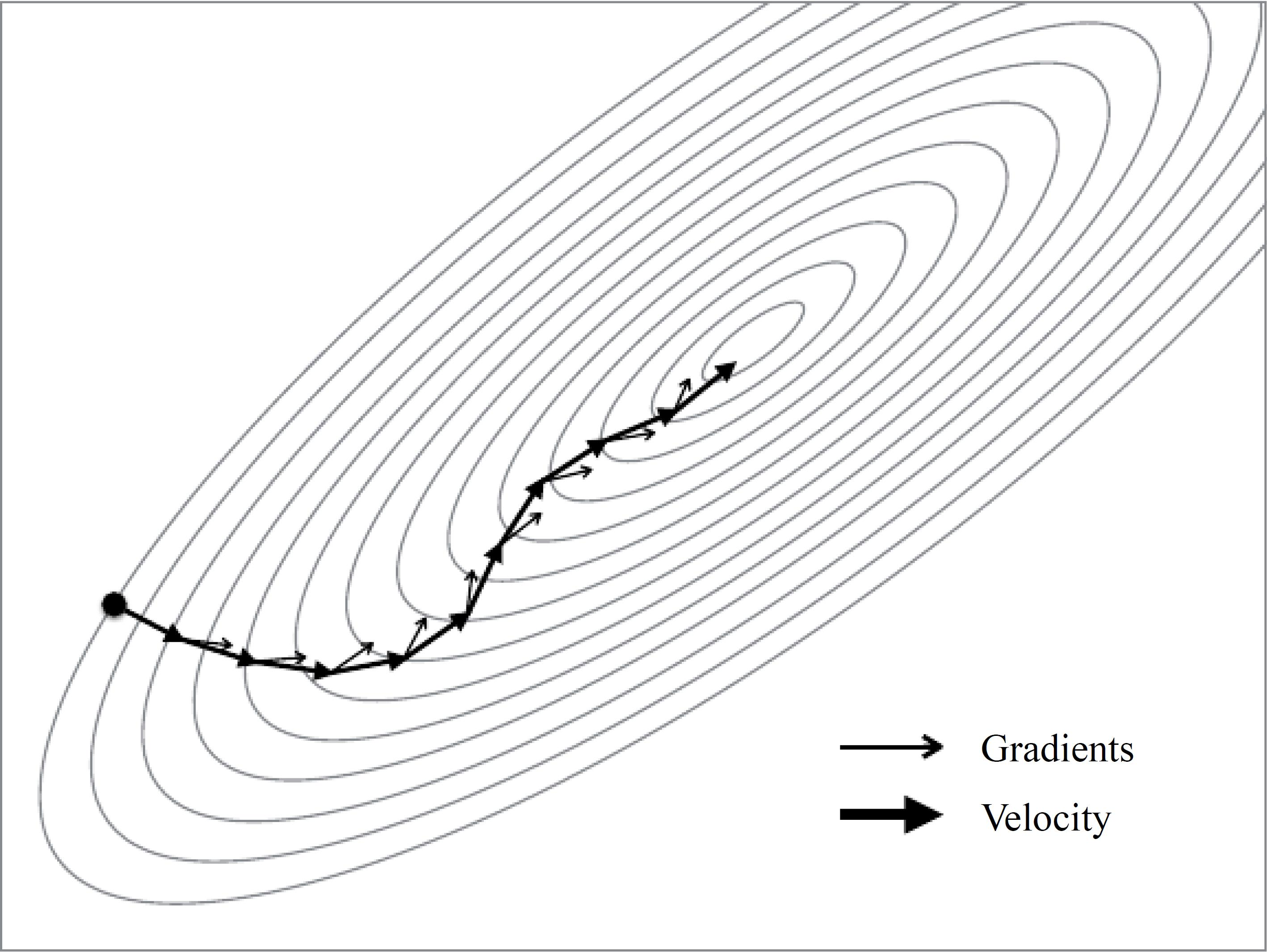Back to our optimization process, we want to accelerate progress along dimensions in which gradient consistently point in the same direction and to slow progress along dimensions where the sign of the gradient continues to change. This is done by keeping track of past parameter updates with an exponential decay:

\begin{align*} \Delta \theta \leftarrow \rho\Delta \theta + \eta g\\ \theta \leftarrow \theta - \Delta \theta \end{align*}

which is mathematically equivalent to

\begin{align*} \Delta \theta \leftarrow \rho\Delta \theta - \eta g\\ \theta \leftarrow \theta + \Delta \theta \end{align*}

where $\rho$ is a constant controlling the decay of the previous parameter updates and $\eta$ is the learning rate. The algorithm is as follows.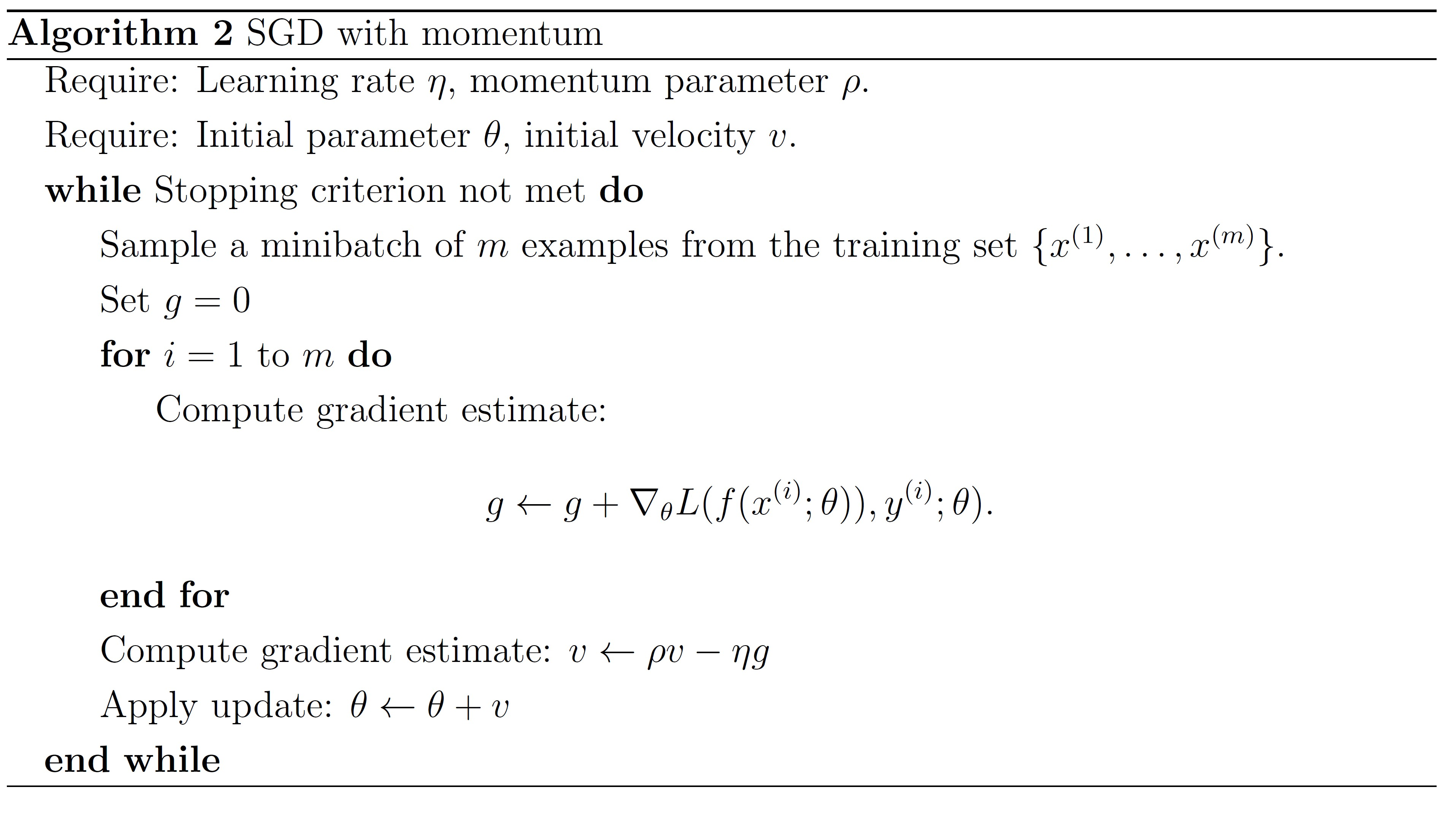This gives a nice improvement over SGD when optimizing difficult cost surfaces. The issue occurred with SGD that a higher learning rate causes oscillations back and forth across the valley has been effectively solved, because the sign of gradient changes and thus the momentum term damps down these updates to slow progress across the valley. And the progress along the valley is unaffected.

## Nesterov Momentum

The standard momentum method first computes the gradient at the current location and then takes a big jump in the direction of the updated accumulated gradient. Ilya  Sutskever (2012 unpublished) suggested a new form of momentum that often works better. The better type of momentum is called Nesterov momentum that first make a big jump in the direction of the previous accumulated gradient, and then measure the gradient where you end up and make a correction. However, in the stochastic gradient case, Nesterov momentum does not improve the rate of convergence.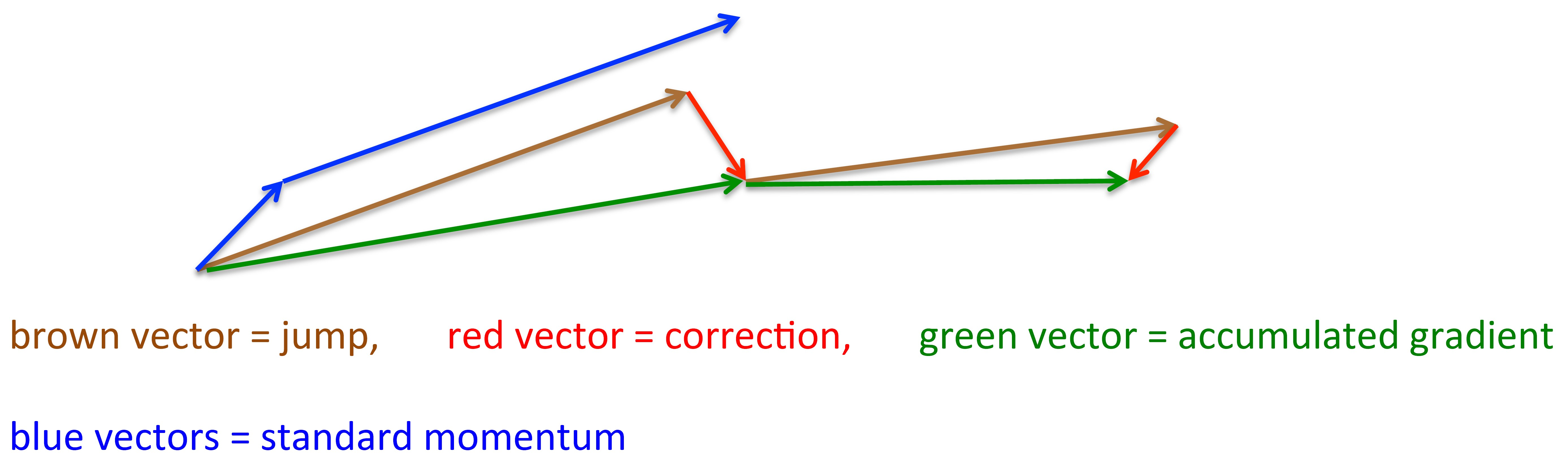The algorithm is as follows.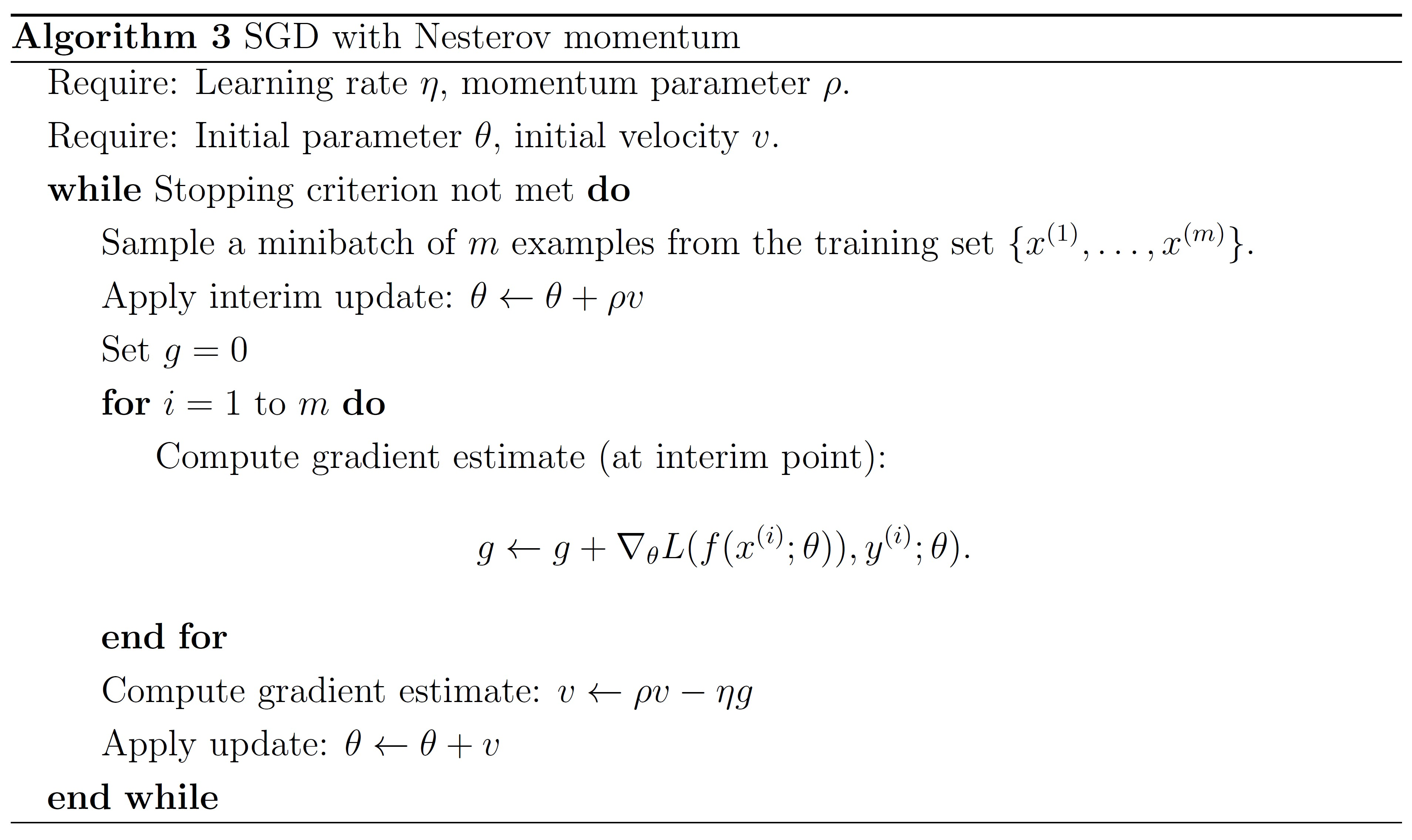Tags: